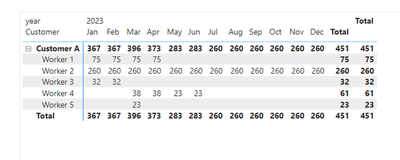cancel
Showing results for
Did you mean:Helper I

## Utilization of resources based on start/end date.

Hello, im trying to calculate utilization rate for resourcers in a company.

The base table looks like this:

 Customer Resource Start Date End Date Customer A Worker 1 02.01.2023 14.04.2023 Customer A Worker 2 02.01.2023 29.12.2023 Customer A Worker 3 16.01.2023 28.02.2023 Customer A Worker 4 01.03.2023 21.04.2023 Customer A Worker 4 05.05.2023 06.06.2023 Customer A Worker 5 01.03.2023 31.03.2023

I've added also column that is calculating working days between start and end date

I would like to calculate utilization for each Worker in a Matrix Visual.

In column I'd like to have year/month in rows there will be Customer/Workers hierarchy and of course values that will be represented as utilization rate so working days between start/end date divided by working days in that month.

I was trying to create something based on this https://www.youtube.com/watch?v=YL7H1Rqckb0 but I believe it should be slightly modified.

Currently Im receiving something like this:and my formula looks like this:

formula =
VAR enddate =
MAX ( 'Calendar'[Date] )
VAR startdate =
MIN ( 'Calendar'[Date] )
VAR result =
CALCULATE (
SUM ( 'Data'[workingdays] ),
ALL ( 'Calendar' ),
'Data'[Start Date] <= enddate,
'Data'[End Date] > startdate
)
RETURN
result

5 REPLIES 5Community Support

``````CountDay =
var _a = MIN('Calendar'[Date])
var _b = MAX('Calendar'[Date])
return SUMX('Table',IF(DATEDIFF(MAXX({_a,[Start Date]},[Value]),MINX({_b,[End Date]},[Value]),DAY)>=0,DATEDIFF(MAXX({_a,[Start Date]},[Value]),MINX({_b,[End Date]},[Value]),DAY)))

Rate =
DIVIDE([CountDay],SUMX('Table',DATEDIFF([Start Date],[End Date],DAY)))``````

Final output:Best Regards,

Jianbo Li

If this post helps, then please consider Accept it as the solution to help the other members find it more quickly.Helper I

Woah 🙂 it loks great, is there any possibility to calculate it only for working days/including holidays? How should I modify the formulaCommunity Support

NETWORKDAYS returns the number of whole workdays between two dates (inclusive). Parameters specify which and how many days are weekend days. Weekend days and days specified as holidays are not considered as workdays. You can try to modify the DAX with NETWORKDAYS function.

Best Regards,

Jianbo Li

If this post helps, then please consider Accept it as the solution to help the other members find it more quickly.Super UserHelper I

Thanks for the links, I see that you are calculating for example count of projects. In my case I would like to present the number of working days for each person in each project monthly. That the actual problem. I was able to present the project count.